Matrix balancing is an interesting problem that has a long history. Matrix balancing refers to adjusting the cells of a frequency table to match known values of the row and column sums. One of the early algorithms for matrix balancing is known as the RAS algorithm, but it is also called the raking algorithm in some fields. The presentation in this article is inspired by a paper by Carol Alderman (1992).

### The problem: Only marginal totals are known

Alderman shows a typical example (p. 83), but let me give a smaller example. Suppose a troop of six Girl Scouts sells seven different types of cookies. At the end of the campaign, each girl reports how many boxes she sold. Also, the troop leader knows how many boxes of each cookie type were sold. However, no one kept track of how many boxes of each type were sold by each girl.

The situation is summarized by the following 7 x 6 table. We know the row totals, which are the numbers of boxes that were sold for each cookie type. We also know the column totals, which are the numbers of boxes that were sold by each girl. But we do not know the values of the individual cells, which are the type-by-girl totals.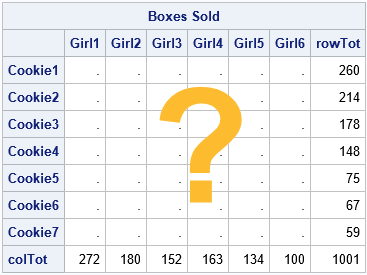### Guessing the cells of a matrix

As stated, the problem usually has infinitely many solutions. For an r x c table, there are r*c cells, but the marginal totals only provide r + c linear constraints. Except for 2 x 2 tables, there are more unknowns than equations, which leads to an underdetermined linear system that (typically) has infinitely many solutions.

Fortunately, often obtain a unique solution if we provide an informed guess for the cells in the table. Perhaps we can ask the girls to provide estimates of the number of boxes of each type that they sold. Or perhaps we have values for the cells from the previous year. In either case, if we can estimate the cell values, we can use a matrix balancing algorithm to adjust the cells so that they match the observed marginal totals.

Let's suppose that the troop leader asks the girls to estimate (from memory) how many boxes of each type they sold. The estimates are shown in the following SAS/IML program. The row and column sums of the estimates do not match the known marginal sums, but that's okay. The program uses subscript reduction operators to compute the marginal sums of the girls' estimates. These are displayed next to the actual totals, along with the differences.

proc iml; /* Known marginal totals: */ u = {260, 214, 178, 148, 75, 67, 59}; /* total boxes sold, by type */ v = {272 180 152 163 134 100}; /* total boxes sold, by girl */   /* We don't know the cell values that produce these marginal totals, but we can ask each girl to estimate how many boxes of each type she sold */ /* G1 G2 G3 G4 G5 G6 */ A = {75 45 40 40 40 30 , /* C1 */ 40 35 45 35 30 30 , /* C2 */ 40 25 30 40 30 20 , /* C3 */ 40 25 25 20 20 20 , /* C4 */ 30 25 0 10 10 0 , /* C5 */ 20 10 10 10 10 0 , /* C6 */ 20 10 0 10 0 0 }; /* C7 */   /* notice that the row/col totals for A do not match the truth: */ rowTotEst = A[ ,+]; /* for each row, sum across all cols */ colTotEst = A[+, ]; /* for each col, sum down all rows */ Items = 'Cookie1':'Cookie7'; /* row labels */ Person = 'Girl1':'Girl6'; /* column labels */   /* print known totals, estimated totals, and difference */ rowSums = (u || rowTotEst || (u-rowTotEst)); print rowSums[r=Items c={'rowTot (u)' 'rowTotEst' 'Diff'}]; colSums = (v // colTotEst // (v-colTotEst)); print colSums[r={'colTot (v)' 'colTotEst' 'Diff'} c=Person];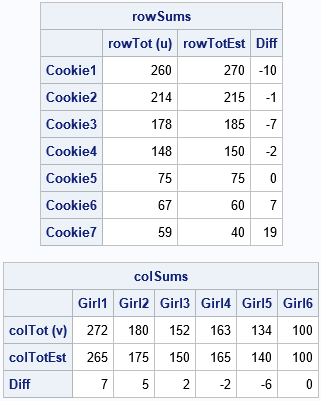You can see that the marginal totals do not match the known row and column totals. However, we can use the guesses as an initial estimate for the RAS algorithm. The RAS algorithm will adjust the estimates to obtain a new matrix that DOES satisfy the marginal totals.

### Adjusting a matrix to match marginal totals

The RAS algorithm can iteratively adjust the girls' estimates until the row sums and column sums of the matrix match the known row sums and column sums. The resulting matrix will probably not be an integer matrix. It will, however, reflect the structure of the girls' estimates in three ways:

1. It will approximately preserve each girl's recollection of the relative proportions of cookie types that she sold. If a girl claims that she sold many of one type of cookie and few of another, that relationship will be reflected in the balanced matrix.
2. It will approximately preserve the relative proportions of cookie types among girls. If one girl claims that she sold many more of one type of cookie than another girl, that relationship will be reflected in the balanced matrix.
3. It will preserve zeros. If a girl claims that she did not sell any of one type of cookie, the balanced matrix will also contain a zero for that cell.

The RAS algorithm is explained in Alderman (1992). The matrix starts with a nonnegative r x c matrix. Let u be the observed r x 1 vector of row sums. Let v be the observed 1 x c vector of column sums. The main steps of the RAS algorithm are:

1. Initialize X = A.
2. Scale the rows. Let R = u / RowSum(X), where 'RowSum' is a function that returns the vector of row sums. Update X ← R # X. Here R#X indicates elementwise multiplication of each column of X by the corresponding element of R.
3. Scale the columns. Let S = v / ColSum(X), where 'ColSum' is a function that returns the vector of column sums. Update X ← X # S. Here X#S indicates elementwise multiplication of each row of X by the corresponding element of S.
4. Check for convergence. If RowSum(X) is close to u and ColSum(X) is close to v, then the algorithm has converged, and X is the balanced matrix. Otherwise, return to Step 2.

The RAS algorithm is implemented in the following SAS/IML program:

/* Use the RAS matrix scaling algorithm to find a matrix X that approximately satisfies X[ ,+] = u (marginals constraints on rows) X[+, ] = v (marginals constraints on cols) and X is obtained by scaling rows and columns of A */ start RAS(A, u, v); X = A; /* Step 0: Initialize X */ converged = 0; do k = 1 to 100 while(^Converged); /* Step 1: Row scaling: X = u # (X / X[ ,+]); */ R = u / X[ ,+]; X = R # X;   /* Step 2: Column scaling: X = (X / X[+, ]) # v; */ S = v / X[+, ]; X = X # S;   /* check for convergence: u=rowTot and v=colTot */ rowTot = X[ ,+]; /* for each row, sum across all cols */ colTot = X[+, ]; /* for each col, sum down all rows */ maxDiff = max( abs(u-rowTot), abs(v-colTot) ); Converged = (maxDiff < 1e-8); end; return( X ); finish;   X = RAS(A, u, v); print X[r=Items c=Person format=7.1];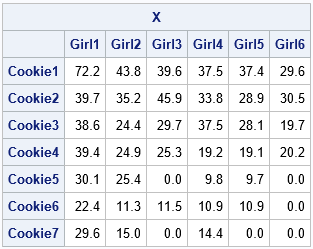The matrix X has row and column sums that are close to the specified values. The matrix also preserves relationships among individual columns and rows, based on the girls' recollections of how many boxes they sold. The algorithm preserves zeros for the girls who claim they sold zero boxes of a certain type. It does not introduce any new zeros for cells.

You can't determine how close the X matrix is to "the truth," because we don't know the true number of boxes that each girl sold. The X matrix depends on the girls' recollections and estimates. If the recollections are close to the truth, then X will be close to the truth as well.

### Summary

This article looks at the RAS algorithm, which is one way to perform matrix balancing on a table that has nonnegative cells. "Balancing" refers to adjusting the interior cells of a matrix to match observed marginal totals. The RAS algorithm starts with an estimate, A. The estimate does not need to satisfy the marginal totals, but it should reflect relationships between the rows and columns that you want to preserve, such as relative proportions and zero cells. The RAS algorithm iteratively scales the rows and columns of A to create a new matrix that matches the observed row and column sums.

It is worth mentioning the row scaling could be accumulated into a diagonal matrix, R, and the column scaling into a diagonal matrix, S. Thus, the balanced matrix is the product of these diagonal matrices and the original estimate, A. As a matrix equation, you can write X = RAS. It is this matrix factorization that gives the RAS algorithm its name.

The RAS is not the only algorithm for matrix balancing, but it is the simplest. Another algorithm, called iterative proportional fitting (IPF), will be discussed in a second article.

The post Matrix balancing: Update matrix cells to match row and column sums appeared first on The DO Loop.On discussion forums, many SAS programmers ask about the best way to generate dummy variables for categorical variables. Well-meaning responders offer all sorts of advice, including writing your own DATA step program, sometimes mixed with macro programming. This article shows that the simplest and easiest way to generate dummy variables in SAS is to use PROC GLMSELECT. It is not necessary to write a SAS program to generate dummy variables. This article shows an example of generating dummy variables that have meaningful names, which are based on the name of the original variable and the categories (levels) of the variable.

A dummy variable is a binary indicator variable. Given a categorical variable, X, that has k levels, you can generate k dummy variables. The j_th dummmy variable indicates the presence (1) or absence (0) of the j_th category.

### Why GLMSELECT is the best way to generate dummy variables

I usually avoid saying "this is the best way" to do something in SAS. But if you are facing an impending deadline, you are probably more interested in solving your problem and less interested in comparing five different ways to solve it. So let's cut to the chase: If you want to generate dummy variables in SAS, use PROC GLMSELECT.

Why do I say that? Because PROC GLMSELECT has the following features that make it easy to use and flexible:

• The syntax of PROC GLMSELECT is straightforward and easy to understand.
• The dummy variables that PROC GLMSELECT creates have meaningful names. For example, if the name of the categorical variable is X and it has values 'A', 'B', and 'C', then the names of the dummy variables are X_A, X_B, and X_C.
• PROC GLMSELECT creates a macro variable named _GLSMOD that contains the names of the dummy variables.
• When you write the dummy variables to a SAS data set, you can include the original variables or not.
• By default, PROC GLMSELECT uses the GLM parameterization of CLASS variables. This is what you need to generate dummy variables. But the same procedure also enables you to generate design matrices that use different parameterizations, that contain interaction effects, that contain spline bases, and more.

The only drawback to using PROC GLMSELECT is that it requires a response variable to put on the MODEL statement. But that is easily addressed.

### How to generate dummy variables

Let's show an example of generating dummy variables. I will use two categorical variables in the Sashelp.Cars data: Origin and Cylinders. First, let's look at the data. As the output from PROC FREQ shows, the Origin variable has three levels ('Asia', 'Europe', and 'USA') and the Cylinders variable has seven valid levels and also contains two missing values.

%let DSIn = Sashelp.Cars; /* name of input data set */ %let VarList = Origin Cylinders; /* name of categorical variables */   proc freq data=&DSIn; tables &VarList; run;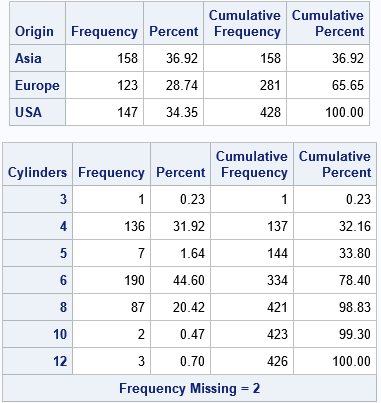In order to use PROC GLMSELECT, you need a numeric response variable. PROC GLMSELECT does not care what the response variable is, but it must exist. The simplest thing to do is to create a "fake" response variable by using a DATA step view. To generate the dummy variables, put the names of the categorical variables on the CLASS and MODEL statements. You can use the OUTDESIGN= option to write the dummy variables (and, optionally, the original variables) to a SAS data set. The following statements generate dummy variables for the Origin and Cylinders variables:

/* An easy way to generate dummy variables is to use PROC GLMSELECT */ /* 1. add a fake response variable */ data AddFakeY / view=AddFakeY; set &DSIn; _Y = 0; run;   /* 2. Create the dummy variables as a GLM design matrix. Include the original variables, if desired */ proc glmselect data=AddFakeY NOPRINT outdesign(addinputvars)=Want(drop=_Y); class &VarList; /* list the categorical variables here */ model _Y = &VarList / noint selection=none; run;

The dummy variables are contained in the WANT data set. As mentioned, the GLMSELECT procedure creates a macro variable (_GLSMOD) that contains the names of the dummy variables. You can use this macro variable in procedures and in the DATA step. For example, you can use it to look at the names and labels for the dummy variables:

/* show the names of the dummy variables */ proc contents varnum data=Want(keep=&_GLSMOD); ods select Position; run;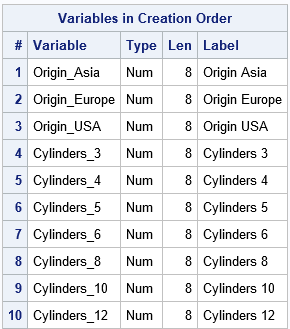Notice that the names of the dummy variables are very understandable. The three levels of the Origin variable are 'Asia', 'Europe', and 'USA, so the dummy variables are named Origin_Asia, Origin_Europe, and Origin_USA. The dummy variables for the seven valid levels of the Cylinders variable are named Cylinders_N, where N is a valid level.

### A macro to generate dummy variables

It is easy to encapsulate the two steps into a SAS macro to make it easier to generate dummy variables. The following statements define the %DummyVars macro, which takes three arguments:

1. DSIn is the name of the input data set, which contains the categorical variables.
2. VarList is a space-separated list of the names of the categorical variables. Dummy variables will be created for each variable that you specify.
3. DSOut is the name of the output data set, which contains the dummy variables.
/* define a macro to create dummy variables */ %macro DummyVars(DSIn, /* the name of the input data set */ VarList, /* the names of the categorical variables */ DSOut); /* the name of the output data set */ /* 1. add a fake response variable */ data AddFakeY / view=AddFakeY; set &DSIn; _Y = 0; /* add a fake response variable */ run; /* 2. Create the design matrix. Include the original variables, if desired */ proc glmselect data=AddFakeY NOPRINT outdesign(addinputvars)=&DSOut(drop=_Y); class &VarList; model _Y = &VarList / noint selection=none; run; %mend;   /* test macro on the Age and Sex variables of the Sashelp.Class data */ %DummyVars(Sashelp.Class, Age Sex, ClassDummy);

When you run the macro, it writes the dummy variables to the ClassDummy data set. It also creates a macro variable (_GLSMOD) that contains the name of the dummy variables. You can use the macro to analyze or print the dummy variables, as follows:

/* _GLSMOD is a macro variable that contains the names of the dummy variables */ proc print data=ClassDummy noobs; var Name &_GLSMod; run;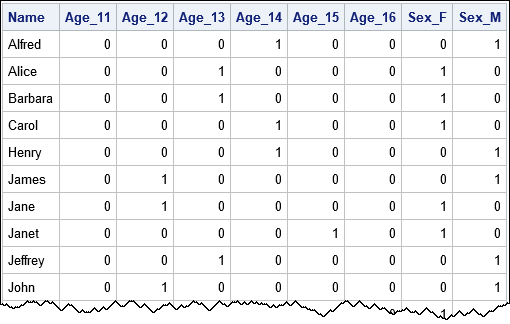The dummy variables tell you that Alfred is a 14-year-old male, Alice is a 13-year-old female, and so forth.

### What happens if a categorical variable contains a missing value?

If a categorical variable contains a missing value, so do all dummy variables that are generated from that variable. For example, we saw earlier that the Cylinders variable for the Sashelp.Cars data has two missing values. You can use PROC MEANS to show that the dummy variables (named Cylinders_N) also have two missing values. Because the dummy variables are binary variables, the sum of each dummy variable matches the number of levels. Compare the SUM column in the PROC MEANS output with the earlier output from PROC FREQ:

/* A missing value in Cylinders results in a missing value for each dummy variable that is generated from Cylinders */ proc means data=Want N NMiss Sum ndec=0; vars Cylinders_:; run;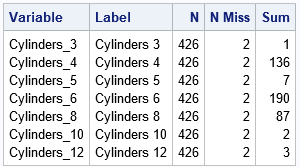### Summary

In most analyses, it is unnecessary to generate dummy variables. Most SAS procedures support the CLASS statement, which enables you to use categorical variables directly in statistical analyses. However, if you do need to generate dummy variables, there is an easy way to do it: Use PROC GENSELECT or use the %DummyVars macro in this article. The result is a SAS data set that contains the dummy variables and a macro variable (_GLSMOD) that contains the names of the dummy variables.

Here are links to previous articles about dummy variables and creating design matrices in SAS.

The post The best way to generate dummy variables in SAS appeared first on The DO Loop.Have you ever seen the "brain teaser" for children that shows a 4 x 4 grid and asks "how many squares of any size are in this grid?" To solve this problem, the reader must recognize that there are sixteen 1 x 1 squares, nine 2 x 2 squares, four 3 x 3 squares, and one 4 x 4 square. Hence there are a total of (16+9+4+1) = 30 squares of any size.

Recently a SAS statistical programmer asked a similar question about submatrices of a matrix. Specifically, the programmer wanted to form square submatrices of consecutive rows and columns. This article shows a few tricks in the SAS/IML language that enable you to generate and compute with all k x k submatrices of a matrix, where the submatrices are formed by using consecutive rows and columns of the original matrix. Although it is possible to consider more general submatrices, in this article, a "submatrix" always refers to one that is formed by using consecutive rows and columns.

### Use subscripts to form submatrices

I've previously written about several ways to extract submatrices from a matrix. Recall the "colon operator" (formally called the index creation operator) generates a vector of consecutive integers. You can use the vectors in the row and column position of the subscripts to extract a submatrix. For example, the following SAS/IML statements define a 4 x 4 matrix and extract the four 3 x 3 submatrices:

A = {4 3 1 6, 2 4 3 1, 0 2 4 3, 5 0 2 4}; /* extract the four 3 x 3 submatrices */ A11 = A[1:3, 1:3]; A12 = A[1:3, 2:4]; A21 = A[2:4, 1:3]; A22 = A[2:4, 2:4];

Manually specifying the row and column numbers is tedious. Let's write a SAS/IML function that will return a k x k submatrix of A by starting at the (i,j)th position of A. Call k the order of the submatrix.

/* Given A, return the square submatrix of order k starting at index (i,j) */ start submat(A, i, j, k); return A[ i:(i+k-1), j:(j+k-1) ]; finish;   /* example : 3 x 3 submatrices of A */ A11 = submat(A, 1, 1, 3); A12 = submat(A, 1, 2, 3); A21 = submat(A, 2, 1, 3); A22 = submat(A, 2, 2, 3);   /* https://blogs.sas.com/content/iml/2015/12/02/matrices-graphs-gridded-layout.html */ ods layout gridded columns=4 advance=table; print A11, A12, A21, A22; ods layout end;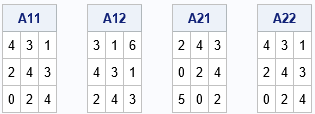### The number of submatrices

If A is an n x m matrix, we can ask how many submatrices of order k are in A. Recall that we are only considering submatrices formed by consecutive rows and columns. There are (n – k + 1) sequences of consecutive rows of length k, such as 1:k, 2:(k+1), and so forth. Similarly, there are (m – k + 1) sequences of consecutive columns of length k. So the following SAS/IML function counts the number of submatrices of order k. You can call the function for different k=1, 2, 3, and 4 in order to solve the "Counting Squares" brain teaser:

/* count all submatrices of order k for the matrix A */ start CountAllSubmat(A, k); numRows = nrow(A)-k+1; numCols = ncol(A)-k+1; return numRows * numCols; finish;   n = nrow(A); numSubmat = j(n, 1, .); do k = 1 to n; numSubmat[k] = CountAllSubmat(A, k); end; order = T(1:n); print order numSubmat;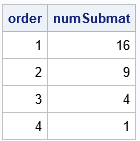If you add up the number of squares each size, you get a total of 30 squares.

### Iterate over submatrices of a specified order

You can iterate over all submatrices of a specified order. You can perform a matrix computation on each submatrix (for example, compute its determinant), or you can pack it into a list for future processing. The following SAS/IML function iterates over submatrices and puts each submatrix into a list. The STRUCT subroutine from the ListUtil package can be used to indicate the contents of the list in a concise form.

start GetListSubmat(A, k); numSub = CountAllSubmat(A, k); L = ListCreate( numSub ); /* create list with numSub items */ cnt = 1; do i = 1 to nrow(A)-k+1; /* for each row */ do j = 1 to ncol(A)-k+1; /* and for each column */ B = submat(A, i, j, k); /* compute submatrix of order k from (i,j)th cell */ call ListSetItem(L, cnt, B); /* put in list (or compute with B here) */ cnt = cnt + 1; end; end; return L; finish;   /* Example: get all matrices of order k=2 */ L = GetListSubmat(A, 2);   package load ListUtil; call Struct(L);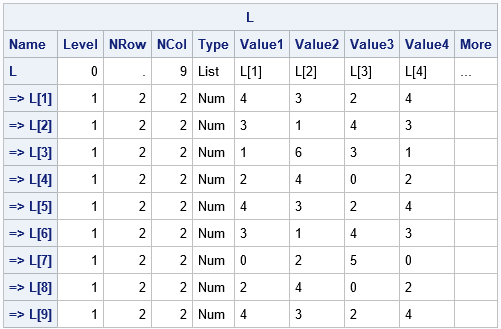The output from the STRUCT call shwos that there are nine 2 x 2 matrices. The Value1–Valuie4 columns show the values (in row-major order). For example, the first 2 x 2 submatrix (in the upper left corner of A) is {4 3, 2 4}. The second submatrix (beginning with A[1,2]) is {3 1, 4 3}.

It is straightforward to modify the function to compute the determinant or some other matrix computation.

### Summary

This article shows some tips and techniques for dealing with submatrices of a matrix. The submatrices in this article are formed by using consecutive rows and columns. Thus, they are similar to the "Counting Squares" brain teaser, which requires that you consider sub-squares of order 1, 2, 3, and so forth.

The post Submatrices of matrices appeared first on The DO Loop.When you write a program that simulates data from a statistical model, you should always check that the simulation code is correct. One way to do this is to generate a large simulated sample, estimate the parameters in the simulated data, and make sure that the estimates are close to the parameters in the simulation. For regression models that include CLASS variables, this task requires a bit of thought. The problem is that categorical variables can have different parameterizations, and the parameterization determines the parameter estimates.

Recently I read a published paper that included a simulation in SAS of data from a logistic model. I noticed that the parameters in the simulation code did not match the parameter estimates in the example. I was initially confused, although I figured it out eventually. This article describes the "parameterization problem" and shows how to ensure that the parameters in the simulation code match the parameter estimates for the analysis.

I have previously written about how to incorporate classification variables into simulations of regression data. If you haven't read that article, it is a good place to begin. You can also read about how to simulate data from a general linear regression model, such as a logistic regression model.

### Simulated explanatory variables with a classification variable

Before demonstrating the problem, let's simulate the explanatory variables. Each simulation will use the same data and the same model but change the way that the model is parameterized. The following SAS DATA step simulates two normal variables and a categorical "treatment" variable that has three levels (Trt=1, 2, or 3). The design is balanced in the treatment variable, which means that each treatment group has the same number of observations.

data SimExplanatory; call streaminit(54321); do i = 1 to 2000; /* number in each treatment group */ do Trt = 1 to 3; /* Trt = 1, 2, and 3 */ x1 = rand('Normal'); /* x1 ~ N(0,1) */ x2 = rand('Normal'); /* x2 ~ N(0,1) */ LinCont = -1.5 + 0.4*x1 - 0.7*x2; /* offset = -1.5; params are 0.4 and -0.7 for x1 and x2 */ output; end; end; drop i; run;

The data set contains a variable called LinCont. The LinCont variable is a linear combination of the x1 and x2 variables and a constant offset. All models will use the same offset (-1.5) and the same parameters for x1 and x2 (0.4 and -0.7, respectively).

### Data for a logistic regression model with a CLASS variable

When writing a simulation of data that satisfy a regression model, most programmers assign coefficients for each level of the treatment variable. For example, you might use the coefficients {1, 2, 3}, which means that the response for the group Trt=1 gets 3 additional units of response, that the group Trt=2 gets 2 additional units, and that the group Trt=3 gets only 1 additional unit. This is a valid way to specify the model, but when you compute the regression estimates, you will discover that they are not close to the parameters in the model. The following DATA step simulates logistic data and uses PROC LOGISTIC to fit a model to the simulated data.

data LogiSim_Attempt; call streaminit(123); set SimExplanatory; /* read the explanatory variables */ array TrtCoef _temporary_ (3, 2, 1); /* treatment effects */ /* a straightforward way to generate Y data from a logistic model */ eta = LinCont + TrtCoef*(Trt=1) + TrtCoef*(Trt=2) + TrtCoef*(Trt=3); p = logistic(eta); /* P(Y | explanatory vars) */ y = rand("Bernoulli", p); /* random realization of Y */ drop eta p; run;   title "Parameter Estimates Do Not Match Simulation Code"; ods select ParameterEstimates; proc logistic data=LogiSim_Attempt plots=none; class Trt; model y(event='1') = Trt x1 x2; run;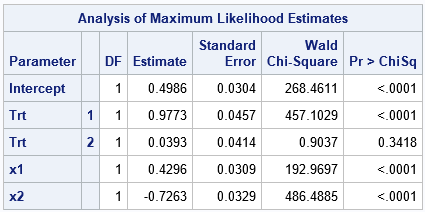Notice that the simulation uses the expression (Trt=1) and (Trt=2) and (Trt=3). These are binary expressions. For any value of Trt, one of the terms is unity and the others are zero.

Notice that the parameter estimates for the levels of the Trt variable do not match the parameters in the model. The model specified parameters {3, 2, 1}, but the estimates are close to {1, 0, ???}, where '???' is used to indicate that the ParameterEstimates table does not include a row for the Trt=3 level. Furthermore, the estimate for the Intercept is not close to the parameter value, which is -1.5.

I see many simulations like this. The problem is that the parameterization used by the statistical analysis procedure (here, PROC LOGISTIC) is different from the model's parameterization. The next section shows how to write a simulation so that the parameters in the simulation code and the parameter estimates agree.

### The 'reference' parameterization

You can't estimate all three coefficients of the categorical variable. However, you can estimate the differences between the levels. For example, you can estimate the average difference between the Trt=1 group and the Trt=3 group. One parameterization of regression models is the "reference parameterization." In the reference parameterization, one of the treatment levels is used as a reference level and you estimate the difference between the non-reference effects and the reference effect. The Intercept parameter is the sum of the offset value and the reference effect. The following simulation code simulates data by using a reference parameterization:

data LogiSim_Ref; call streaminit(123); set SimExplanatory; /* assume reference or GLM parameterization */ array TrtCoef _temporary_ (3, 2, 1); /* treatment effects */ RefLevel = TrtCoef; /* use last level as reference */ eta = LinCont + RefLevel /* constant term is (Intercept + RefLevel) */ + (TrtCoef-RefLevel)*(Trt=1) /* param = +2 */ + (TrtCoef-RefLevel)*(Trt=2) /* param = +1 */ + (TrtCoef-RefLevel)*(Trt=3); /* param = 0 */ p = logistic(eta); /* P(Y | explanatory vars) */ y = rand("Bernoulli", p); /* random realization of Y */ drop eta p; run;   title "Reference Parameterization"; ods select ParameterEstimates; proc logistic data=LogiSim_Ref plots=none; class Trt / param=reference ref=LAST; /* reference parameterization */ model y(event='1') = Trt x1 x2; run;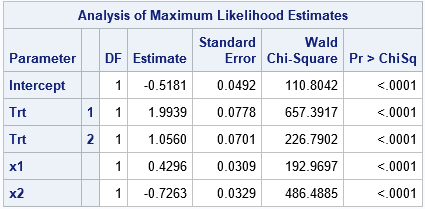In this simulation and analysis, the constant term in the model is "Intercept + RefLevel," which is -0.5. The parameter for the Trt=1 effect is +2, which is "TrtCoef-RefLevel." Similarly, the parameter for the Trt=2 effect is +1, and the parameter for Trt=3 is 0. Notice that the parameter estimates from PROC LOGISTIC agree with these values because I specified the PARAM=REFERENCE option, which tells the procedure to use the same parameterization as the simulation code.

Notice that this model is the same as the previous model because we are simply adding the RefLevel to the constant term and subtracting it from the Trt effect.

### The 'effect' parameterization

In a similar way, instead of subtracting a reference level, you can subtract the mean of the coefficients for the treatment effects. In general, you need to compute a weighted mean, where the weights are the number of observations for each level, but for the case of balanced treatments, you can just use the ordinary arithmetic mean of the coefficients.

For these data, the mean of the treatment effects is (3+2+1)/3 = 2. The following simulation adds the mean to the constant term and subtracts it from the Trt effects. This is the "effect parameterization":

data LogiSim_Effect; call streaminit(123); set SimExplanatory; array TrtCoef _temporary_ (3, 2, 1); /* treatment effects */ /* assume effect parameterization, which is equivalent to using deviations from the mean: array TrtDev _temporary_ (+1, 0, -1); */   MeanTrt = 2; /* for balanced design, mean=(3+2+1)/3 */ eta = LinCont + MeanTrt /* est intercept = (Intercept + MeanTrt) */ + (TrtCoef-MeanTrt)*(Trt=1) /* param = +1 */ + (TrtCoef-MeanTrt)*(Trt=2) /* param = 0 */ + (TrtCoef-MeanTrt)*(Trt=3); /* param = -1 */ p = logistic(eta); /* P(Y | explanatory vars) */ y = rand("Bernoulli", p); /* random realization of Y */ drop eta p; run;   title "Effect Parameterization"; ods select ParameterEstimates; proc logistic data=LogiSim_effect plots=none; class Trt / param=effect ref=LAST; model y(event='1') = Trt x1 x2; run;In this simulation and analysis, the constant term in the model is "Intercept + MeanTrt," which is 0.5. The parameters for the Trt levels are {+1, 0, -1}. Notice that the PROC LOGISTIC call uses the PARAM=EFFECT option, which tells the procedure to use the same effect parameterization. Consequently, the parameter estimates are close to the parameter values. (These are the same parameter estimates as in the first example, since PROC LOGISTIC uses the effect parameterization by default.)

If you use 0 as an offset value, then the Intercept parameter equals the mean effect of the treatment variable, which leads to a nice interpretation when there is only one classification variable and no interactions.

### Summary

This article shows how to write the simulation code (the equation of the linear predictor) so that it matches the parameterization used to analyze the data. This article uses PROC LOGISTIC, but you can use the same trick for other procedures and analyses. When the simulation code and the analysis use the same parameterization of classification effects, it is easier to feel confident that your simulation code correctly simulates data from the model that you are analyzing.

The post Simulate regression models that incorporate CLASS parameterizations appeared first on The DO Loop.When you write a program that simulates data from a statistical model, you should always check that the simulation code is correct. One way to do this is to generate a large simulated sample, estimate the parameters in the simulated data, and make sure that the estimates are close to the parameters in the simulation. For regression models that include CLASS variables, this task requires a bit of thought. The problem is that categorical variables can have different parameterizations, and the parameterization determines the parameter estimates.

Recently I read a published paper that included a simulation in SAS of data from a logistic model. I noticed that the parameters in the simulation code did not match the parameter estimates in the example. I was initially confused, although I figured it out eventually. This article describes the "parameterization problem" and shows how to ensure that the parameters in the simulation code match the parameter estimates for the analysis.

I have previously written about how to incorporate classification variables into simulations of regression data. If you haven't read that article, it is a good place to begin. You can also read about how to simulate data from a general linear regression model, such as a logistic regression model.

### Simulated explanatory variables with a classification variable

Before demonstrating the problem, let's simulate the explanatory variables. Each simulation will use the same data and the same model but change the way that the model is parameterized. The following SAS DATA step simulates two normal variables and a categorical "treatment" variable that has three levels (Trt=1, 2, or 3). The design is balanced in the treatment variable, which means that each treatment group has the same number of observations.

data SimExplanatory; call streaminit(54321); do i = 1 to 2000; /* number in each treatment group */ do Trt = 1 to 3; /* Trt = 1, 2, and 3 */ x1 = rand('Normal'); /* x1 ~ N(0,1) */ x2 = rand('Normal'); /* x2 ~ N(0,1) */ LinCont = -1.5 + 0.4*x1 - 0.7*x2; /* offset = -1.5; params are 0.4 and -0.7 for x1 and x2 */ output; end; end; drop i; run;

The data set contains a variable called LinCont. The LinCont variable is a linear combination of the x1 and x2 variables and a constant offset. All models will use the same offset (-1.5) and the same parameters for x1 and x2 (0.4 and -0.7, respectively).

### Data for a logistic regression model with a CLASS variable

When writing a simulation of data that satisfy a regression model, most programmers assign coefficients for each level of the treatment variable. For example, you might use the coefficients {1, 2, 3}, which means that the response for the group Trt=1 gets 3 additional units of response, that the group Trt=2 gets 2 additional units, and that the group Trt=3 gets only 1 additional unit. This is a valid way to specify the model, but when you compute the regression estimates, you will discover that they are not close to the parameters in the model. The following DATA step simulates logistic data and uses PROC LOGISTIC to fit a model to the simulated data.

data LogiSim_Attempt; call streaminit(123); set SimExplanatory; /* read the explanatory variables */ array TrtCoef _temporary_ (3, 2, 1); /* treatment effects */ /* a straightforward way to generate Y data from a logistic model */ eta = LinCont + TrtCoef*(Trt=1) + TrtCoef*(Trt=2) + TrtCoef*(Trt=3); p = logistic(eta); /* P(Y | explanatory vars) */ y = rand("Bernoulli", p); /* random realization of Y */ drop eta p; run;   title "Parameter Estimates Do Not Match Simulation Code"; ods select ParameterEstimates; proc logistic data=LogiSim_Attempt plots=none; class Trt; model y(event='1') = Trt x1 x2; run;Notice that the simulation uses the expression (Trt=1) and (Trt=2) and (Trt=3). These are binary expressions. For any value of Trt, one of the terms is unity and the others are zero.

Notice that the parameter estimates for the levels of the Trt variable do not match the parameters in the model. The model specified parameters {3, 2, 1}, but the estimates are close to {1, 0, ???}, where '???' is used to indicate that the ParameterEstimates table does not include a row for the Trt=3 level. Furthermore, the estimate for the Intercept is not close to the parameter value, which is -1.5.

I see many simulations like this. The problem is that the parameterization used by the statistical analysis procedure (here, PROC LOGISTIC) is different from the model's parameterization. The next section shows how to write a simulation so that the parameters in the simulation code and the parameter estimates agree.

### The 'reference' parameterization

You can't estimate all three coefficients of the categorical variable. However, you can estimate the differences between the levels. For example, you can estimate the average difference between the Trt=1 group and the Trt=3 group. One parameterization of regression models is the "reference parameterization." In the reference parameterization, one of the treatment levels is used as a reference level and you estimate the difference between the non-reference effects and the reference effect. The Intercept parameter is the sum of the offset value and the reference effect. The following simulation code simulates data by using a reference parameterization:

data LogiSim_Ref; call streaminit(123); set SimExplanatory; /* assume reference or GLM parameterization */ array TrtCoef _temporary_ (3, 2, 1); /* treatment effects */ RefLevel = TrtCoef; /* use last level as reference */ eta = LinCont + RefLevel /* constant term is (Intercept + RefLevel) */ + (TrtCoef-RefLevel)*(Trt=1) /* param = +2 */ + (TrtCoef-RefLevel)*(Trt=2) /* param = +1 */ + (TrtCoef-RefLevel)*(Trt=3); /* param = 0 */ p = logistic(eta); /* P(Y | explanatory vars) */ y = rand("Bernoulli", p); /* random realization of Y */ drop eta p; run;   title "Reference Parameterization"; ods select ParameterEstimates; proc logistic data=LogiSim_Ref plots=none; class Trt / param=reference ref=LAST; /* reference parameterization */ model y(event='1') = Trt x1 x2; run;In this simulation and analysis, the constant term in the model is "Intercept + RefLevel," which is -0.5. The parameter for the Trt=1 effect is +2, which is "TrtCoef-RefLevel." Similarly, the parameter for the Trt=2 effect is +1, and the parameter for Trt=3 is 0. Notice that the parameter estimates from PROC LOGISTIC agree with these values because I specified the PARAM=REFERENCE option, which tells the procedure to use the same parameterization as the simulation code.

Notice that this model is the same as the previous model because we are simply adding the RefLevel to the constant term and subtracting it from the Trt effect.

### The 'effect' parameterization

In a similar way, instead of subtracting a reference level, you can subtract the mean of the coefficients for the treatment effects. In general, you need to compute a weighted mean, where the weights are the number of observations for each level, but for the case of balanced treatments, you can just use the ordinary arithmetic mean of the coefficients.

For these data, the mean of the treatment effects is (3+2+1)/3 = 2. The following simulation adds the mean to the constant term and subtracts it from the Trt effects. This is the "effect parameterization":

data LogiSim_Effect; call streaminit(123); set SimExplanatory; array TrtCoef _temporary_ (3, 2, 1); /* treatment effects */ /* assume effect parameterization, which is equivalent to using deviations from the mean: array TrtDev _temporary_ (+1, 0, -1); */   MeanTrt = 2; /* for balanced design, mean=(3+2+1)/3 */ eta = LinCont + MeanTrt /* est intercept = (Intercept + MeanTrt) */ + (TrtCoef-MeanTrt)*(Trt=1) /* param = +1 */ + (TrtCoef-MeanTrt)*(Trt=2) /* param = 0 */ + (TrtCoef-MeanTrt)*(Trt=3); /* param = -1 */ p = logistic(eta); /* P(Y | explanatory vars) */ y = rand("Bernoulli", p); /* random realization of Y */ drop eta p; run;   title "Effect Parameterization"; ods select ParameterEstimates; proc logistic data=LogiSim_effect plots=none; class Trt / param=effect ref=LAST; model y(event='1') = Trt x1 x2; run;In this simulation and analysis, the constant term in the model is "Intercept + MeanTrt," which is 0.5. The parameters for the Trt levels are {+1, 0, -1}. Notice that the PROC LOGISTIC call uses the PARAM=EFFECT option, which tells the procedure to use the same effect parameterization. Consequently, the parameter estimates are close to the parameter values. (These are the same parameter estimates as in the first example, since PROC LOGISTIC uses the effect parameterization by default.)

If you use 0 as an offset value, then the Intercept parameter equals the mean effect of the treatment variable, which leads to a nice interpretation when there is only one classification variable and no interactions.

### Summary

This article shows how to write the simulation code (the equation of the linear predictor) so that it matches the parameterization used to analyze the data. This article uses PROC LOGISTIC, but you can use the same trick for other procedures and analyses. When the simulation code and the analysis use the same parameterization of classification effects, it is easier to feel confident that your simulation code correctly simulates data from the model that you are analyzing.

The post Simulate regression models that incorporate CLASS parameterizations appeared first on The DO Loop.Last month a SAS programmer asked how to fit a multivariate Gaussian mixture model in SAS. For univariate data, you can use the FMM Procedure, which fits a large variety of finite mixture models. If your company is using SAS Viya, you can use the MBC or GMM procedures, which perform model-based clustering (PROC MBC) or cluster analysis by using the Gaussian mixture model (PROC GMM). The MBC procedure is part of SAS Visual Statistics; The GMM procedure is part of SAS Visual Data Mining and Machine Learning (VDMML).

### The problem: Fitting a Gaussian mixture model

A Gaussian mixture model assumes that each cluster is multivariate normal but allows different clusters to have different within-cluster covariance structures. As in k-means clustering, it is assumed that you know the number of clusters, G. The clustering problem is an "unsupervised" machine learning problem, which means that the observations do not initially have labels that tell you which observation belongs to which cluster. The goal of the analysis is to assign each observation to a cluster ("hard clustering") or a probability of belonging to each cluster ("fuzzy clustering"). I will use the terms "cluster" and "group" interchangeably. In this article, I will only discuss the hard-clustering problem, which is conceptually easier to understand and implement.

The density of a Gaussian mixture with G components is $\Sigma_{i=1}^G \tau_i f(\mathbf{x}; {\boldsymbol\mu}_i, {\boldsymbol\Sigma}_i)$, where f(x; μi, Σi) is the multivariate normal density of the i_th component, which has mean vector μi and covariance matrix Σi. The values τi are the mixing probabilities, so Σ τi = 1. If x is a d-dimensional vector, you need to estimate τi, μi, and Σi for each of the G groups, for a total of G*(1 + d + d*(d-1)/2) – 1 parameter estimates. The d*(d-1)/2 expression is the number of free parameters in the symmetric covariance matrix and the -1 term reflects the constraint Σ τi = 1. Fortunately, the assumption that the groups are multivariate normal enables you to compute the maximum likelihood estimates directly without doing any numerical optimization.

Fitting a Gaussian mixture model is a "chicken-and-egg" problem because it consists of two subproblems, each of which is easy to solve if you know the answer to the other problem:

• If you know to which group each observation belongs, you can compute maximum likelihood estimates for the mean (center) and covariance of each group. You can also use the number of observations in each group to estimate the mixing probabilities for the finite mixture distribution.
• If you know the center, covariance matrix, and mixing probabilities for each of the G groups, you can use the density function for each group (weighted by the mixing probability) to determine how likely each observation is to belong to a cluster. For "hard clustering," you assign the observation to the cluster that gives the highest likelihood.

The expectation-maximization (EM) algorithm is an iterative method that enables you to solve interconnected problems like this. The steps of the EM algorithm are given in the documentation for the MBC procedure, as follows:

1. Use some method (such as k-means clustering) to assign each observation to a cluster. The assignment does not have to be precise because it will be refined. Some data scientists use random assignment as a quick-and-dirty way to initially assign points to clusters, but for hard clustering this can lead to less than optimal solutions.
2. The M Step: Assume that the current assignment to clusters is correct. Compute the maximum likelihood estimates of the within-cluster count, mean, and covariance. From the counts, estimate the mixing probabilities. I have previously shown how to compute the within-group parameter estimates
3. The E Step: Assume the within-cluster statistics are correct. I have previously shown how to evaluate the likelihood that each observation belongs to each cluster. Use the likelihoods to update the assignment to clusters. For hard clustering, this means choosing the cluster for which the density (weighted by the mixing probabilities) is greatest.
4. Evaluate the mixture log likelihood, which is an overall measure of the goodness of fit. If the log likelihood has barely changed from the previous iteration, assume that the EM algorithm has converged. Otherwise, go to the M step and repeat until convergence. The documentation for PROC MBC provides the log-likelihood function for both hard and fuzzy clustering.

This implementation of the EM algorithm performs the M-step before the E-step, but it is still the "EM" algorithm. (Why? Because there is no "ME" in statistics!)

### Use k-means clustering to assign the initial group membership

The first step is to assign each observation to a cluster. Let's use the Fisher Iris data, which we know has three clusters. We will not use the Species variable in any way, but merely treat the data as four-dimensional unlabeled data.

The following steps are adapted from the Getting Started example in PROC FASTCLUS. The call to PROC STDIZE standardizes the data and the call to PROC FASTCLUS performs k-means clustering and saves the clusters to an output data set.

/* standardize and use k-means clustering (k=3) for initial guess */ proc stdize data=Sashelp.Iris out=StdIris method=std; var Sepal: Petal:; run;   proc fastclus data=StdIris out=Clust maxclusters=3 maxiter=100 random=123; var Sepal: Petal:; run;   data Iris; merge Sashelp.Iris Clust(keep=Cluster); /* for consistency with the Species order, remap the cluster numbers */ if Cluster=1 then Cluster=2; else if Cluster=2 then Cluster=1; run;   title "k-Means Clustering of Iris Data"; proc sgplot data=Iris; ellipse x=PetalWidth y=SepalWidth / group=Cluster; scatter x=PetalWidth y=SepalWidth / group=Cluster transparency=0.2 markerattrs=(symbol=CircleFilled size=10) jitter; xaxis grid; yaxis grid; run;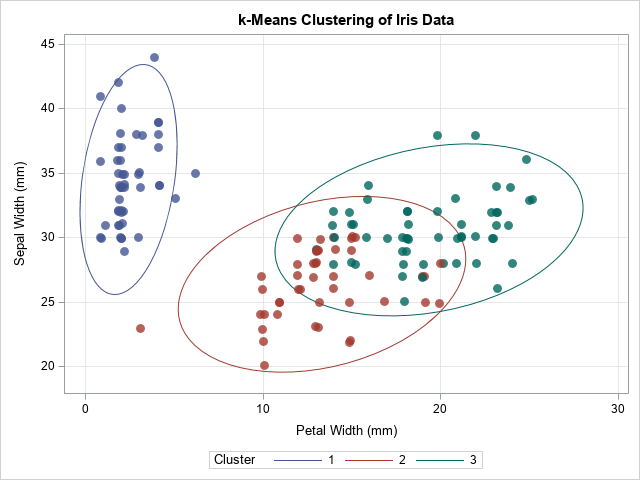The graph shows the cluster assignments from PROC FASTCLUS. They are similar but not identical to the actual groups of the Species variable. In the next section, these cluster assignments are used to initialize the EM algorithm.

### The EM algorithm for hard clustering

You can write a SAS/IML program that implements the EM algorithm for hard clustering. The M step uses the MLEstMVN function (described in a previous article) to compute the maximum likelihood estimates within clusters. The E step uses the LogPdfMVN function (described in a previous article) to compute the log-PDF for each cluster (assuming MVN).

proc iml; load module=(LogPdfMVN MLEstMVN); /* you need to STORE these modules */   /* 1. Read data. Initialize 'Cluster' assignments from PROC FASTCLUS */ use Iris; varNames = {'SepalLength' 'SepalWidth' 'PetalLength' 'PetalWidth'}; read all var varNames into X; read all var {'Cluster'} into Group; /* from PROC FASTCLUS */ close;   nobs = nrow(X); d = ncol(X); G = ncol(unique(Group)); prevCDLL = -constant('BIG'); /* set to large negative number */ converged = 0; /* iterate until converged=1 */ eps = 1e-5; /* convergence criterion */ iterHist = j(100, 3, .); /* monitor EM iteration */ LL = J(nobs, G, 0); /* store the LL for each group */   /* EM algorithm: Solve the M and E subproblems until convergence */ do nIter = 1 to 100 while(^converged); /* 2. M Step: Given groups, find MLE for n, mean, and cov within each group */ L = MLEstMVN(X, Group); /* function returns a list */ ns = L$'n'; tau = ns / sum(ns); means = L$'mean'; covs = L$'cov'; /* 3. E Step: Given MLE estimates, compute the LL for membership in each group. Use LL to update group membership. */ do k = 1 to G; LL[ ,k] = log(tau[k]) + LogPDFMVN(X, means[k,], shape(covs[k,],d)); end; Group = LL[ ,<:>]; /* predicted group has maximum LL */ /* 4. The complete data LL is the sum of log(tau[k]*PDF[,k]). For "hard" clustering, Z matrix is 0/1 indicator matrix. DESIGN function: https://blogs.sas.com/content/iml/2016/03/02/dummy-variables-sasiml.html */ Z = design(Group); /* get dummy variables for Group */ CDLL = sum(Z # LL); /* sum of LL weighted by group membership */ /* compute the relative change in CD LL. Has algorithm converged? */ relDiff = abs( (prevCDLL-CDLL) / prevCDLL ); converged = ( relDiff < eps ); /* monitor convergence; if no convergence, iterate */ prevCDLL = CDLL; iterHist[nIter,] = nIter || CDLL || relDiff; end; /* remove unused rows and print EM iteration history */ iterHist = iterHist[ loc(iterHist[,2]^=.), ]; print iterHist[c={'Iter' 'CD LL' 'relDiff'}];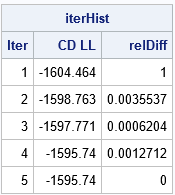The output from the iteration history shows that the EM algorithm converged in five iterations. At each step of the iteration, the log likelihood increased, which shows that the fit of the Gaussian mixture model improved at each iteration. This is one of the features of the EM algorithm: the likelihood always increases on successive steps. ### The results of the EM algorithm for fitting a Gaussian mixture model This problem uses G=3 clusters and d=4 dimensions, so there are 3*(1 + 4 + 4*3/2) – 1 = 32 parameter estimates! Most of those parameters are the elements of the three symmetric 4 x 4 covariance matrices. The following statements print the estimates of the mixing probabilities, the mean vector, and the covariance matrices for each cluster. To save space, the covariance matrices are flattened into a 16-element row vector. /* print final parameter estimates for Gaussian mixture */ GroupNames = strip(char(1:G)); rows = repeat(T(1:d), 1, d); cols = repeat(1:d, d, 1); SNames = compress('S[' + char(rows) + ',' + char(cols) + ']'); print tau[r=GroupNames F=6.2], means[r=GroupNames c=varNames F=6.2], covs[r=GroupNames c=(rowvec(SNames)) F=6.2];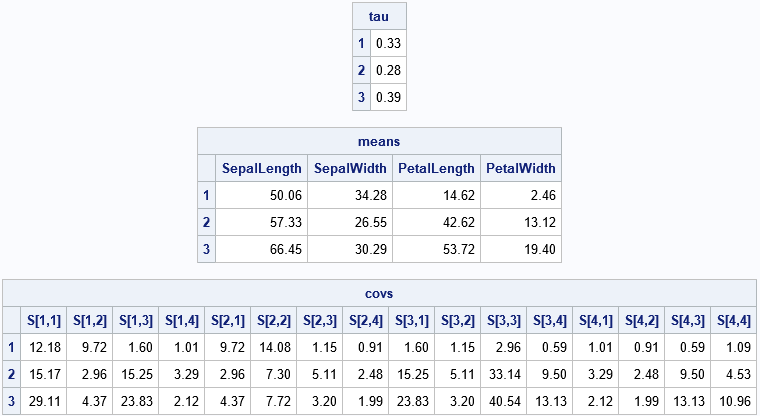You can merge the final Group assignment with the data and create scatter plots that show the group assignment. One of the scatter plots is shown below: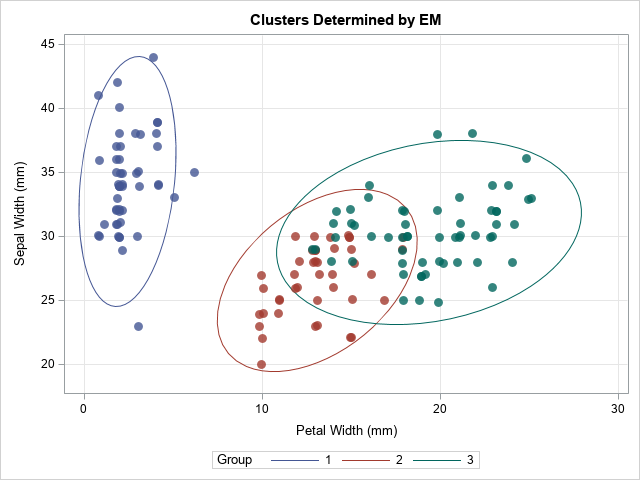The EM algorithm changed the group assignments for 10 observations. The most obvious change is the outlying marker in the lower-left corner, which changed from Group=2 to Group=1. The other markers that changed are near the overlapping region between Group=2 and Group=3. ### Summary This article shows an implementation of the EM algorithm for fitting a Gaussian mixture model. For simplicity, I implemented an algorithm that uses hard clustering (the complete data likelihood model). This algorithm might not perform well with a random initial assignment of clusters, so I used the results of k-means clustering (PROC FASTCLUS) to initialize the algorithm. Hopefully, some of the tricks and techniques in this implementation of the EM algorithm will be useful to SAS programmers who want to write more sophisticated EM programs. The main purpose of this article is to demonstrate how to implement the EM algorithm in SAS/IML. As a reminder, if you want to fit a Gaussian mixture model in SAS, you can use PROC MBC or PROC GMM in SAS Viya. The MBC procedure is especially powerful: in Visual Statistics 8.5 you can choose from 17 different ways to model the covariance structure in the clusters. The MBC procedure also supports several ways to initialize the group assignments and supports both hard and fuzzy clustering. For more about the MBC procedure, see Kessler (2019), "Introducing the MBC Procedure for Model-Based Clustering." I learned a lot by writing this program, so I hope it is helpful. You can download the complete SAS program that implements the EM algorithm for fitting a Gaussian mixture model. Leave a comment if you find it useful.I recently showed how to compute within-group multivariate statistics by using the SAS/IML language. However, a principal of good software design is to encapsulate functionality and write self-contained functions that compute and return the results. What is the best way to return multiple statistics from a SAS/IML module? A convenient way to return several statistics is to use lists. Lists were introduced in SAS/IML 14.2 and extended in SAS/IML 14.3. I have previously written about how to use lists to pass arguments into a SAS/IML module. For parallel processing in the iml action in SAS Viya, lists are an essential data structure. This article shows two things. First, it shows how to use the UNIQUE-LOC technique to compute multivariate statistics for each group in the data. It then shows two ways to return those statistics by using SAS/IML lists. Depending on your application, one way might be more convenient to use than the other. ### Returning matrices versus lists Suppose you want to write a SAS/IML function that computes several statistics for each group in the data. If you are analyzing one variable (univariate data), the statistics are scalar. Therefore, the module can return a matrix in which the rows of the matrix represent the groups in the data and the columns represent the statistics, such as mean, median, variance, skewness, and so forth. This form of the output matches the output from PROC MEANS when you use a CLASS statement to specify the group variable. For multivariate data, the situation becomes more complex. For example, suppose that for each group you want to compute the number of observations, the mean vector, and the covariance matrix. Although it is possible to pack all this information into a matrix, it requires a lot of bookkeeping to keep track of which row contains which statistics for which group. A simpler method is to pack the multivariate statistics into a list and return the list. ### Multivariate statistics for the iris data Fisher's iris data is often used to demonstrate ideas in multivariate statistics. The following call to PROC SGPLOT creates a scatter plot for two variables (PetalWidth and SepalLength) and uses the GROUP= option to color the observations by the levels of the Species variable. The plot also overlays 95% prediction ellipses for each group. title "Iris Data, Colored by Species"; title2 "95% Prediction Ellipse, assuming MVN"; proc sgplot data=Sashelp.Iris; ellipse x=PetalWidth y=SepalWidth / group=Species outline fill transparency=0.6; scatter x=PetalWidth y=SepalWidth / group=Species transparency=0.2 markerattrs=(symbol=CircleFilled size=10) jitter; xaxis grid; yaxis grid; run;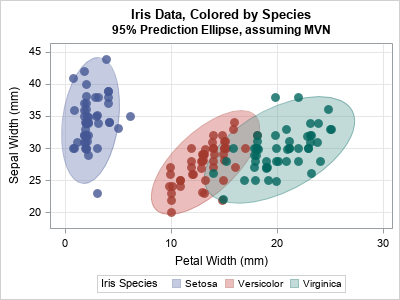Although PROC SGPLOT computes these ellipses automatically, you might be interested in the multivariate statistics behind the ellipses. The ellipses depend on the number of observations, the mean vector, and the covariance matrix for each group. The following SAS/IML module computes these quantities for each group. The module assembles the statistics into three matrices: • The ns vector contains the number of observations. The k_th row contains the number of observations in the k_th group. • The means matrix contains the group means. The k_th row contains the mean vector for the k_th group. • The covs matrix contains the group covariance matrices. The k_th row contains the covariance matrix for the k_th group. You can use the ROWVEC function to "flatten" a matrix into a row vector. When you want to reconstruct the matrix, you can use the SHAPE function, as discussed in the article "Create an array of matrices in SAS." These three items are packed into a list and returned by the module. proc iml; /* GIVEN Y and a classification variable Group that indicate group membership, return a list that contains: 1. Number of observations in each group 2. ML estimates of within-group mean 3. ML estimate of within-group covariance The k_th row of each matrix contains the statistics for the k_th group. */ start MLEstMVN(Y, Group); d = ncol(Y); u = unique(Group); G = ncol(u); ns = J(G, 1, 0); /* k_th row is number of obs in k_th group */ means = J(G, d, 0); /* k_th row is mean of k_th group */ covs = J(G, d*d, 0) ; /* k_th row is cov of k_th group */ do k = 1 to G; X = Y[loc(Group=u[k]), ]; /* obs in k_th group */ n = nrow(X); /* number of obs in this group */ C = (n-1)/n * cov(X); /* ML estimate of COV does not use the Bessel correction */ ns[k] = n; means[k, ] = mean(X); /* ML estimate of mean */ covs[k, ] = rowvec( C ); /* store COV in k_th row */ end; outList = [#'n'=ns, #'mean'=means, #'cov'=Covs, #'group'=u]; /* pack into list */ return (outList); finish; /* Example: read the iris data */ varNames = {'PetalWidth' 'SepalWidth'}; use Sashelp.Iris; read all var varNames into X; read all var {'Species'}; close; L = MLEstMVN(X, Species); /* call function; return named list of results */ ns = L$'n'; /* extract obs numbers */ means = L$'mean'; /* extract mean vectors */ Covs = L$'cov'; /* extract covariance matrix */ lbl = L$'group'; print ns[r=lbl c='n'], means[r=lbl c={'m1' 'm2'}], covs[r=lbl c={C11 C12 C21 C22}];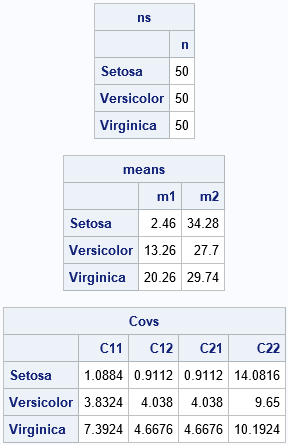The multivariate statistics are shown. The k_th row of each matrix contains a statistic for the k_th group. The number of observations and the mean vectors are easy to understand and interpret. The covariance matrix for each group has been flattened into a row vector. You can use the SHAPE function to rearrange the row into a matrix. For example, the covariance matrix for the first group ('Setosa') can be constructed as Setosa_Cov = shape(covs[1,], 2). ### An alternative: A list of lists It can be convenient to return a list of lists that contains the statistics. Suppose you want to compute m statistics for each of G groups. The function in the previous section returned a list that contains m items and each item had G rows. (I also included an additional list item, the group levels, but that is optional.) Alternatively, you could return a list that has G sublists, where each sublist contains the m statistics for the group. This is shown in the following function, which returns the same information but organizes it in a different way. /* Alternative: Return a list of G sublists. Each sublist has only the statistics relevant to the corresponding group. */ start MLEst2(Y, Group); u = unique(Group); G = ncol(u); outList = ListCreate(G); do k = 1 to G; X = Y[loc(Group=u[k]), ]; /* obs in k_th group */ n = nrow(X); /* number of obs in k_th group */ SL = [#'n'=n, #'mean'=mean(X), /* ML estimate of mean */ #'cov'=(n-1)/n*cov(X)]; /* ML estimate of COV; no need to flatten */ call ListSetItem(outList, k, SL); /* set sublist as the k_th item */ end; call ListSetName(outList, 1:G, u); /* give a name to each sublist */ return (outList); finish; L2 = MLEst2(X, Species); package load ListUtil; /* load a package to print lists */ call Struct(L2); /* or: call ListPrint(L2); */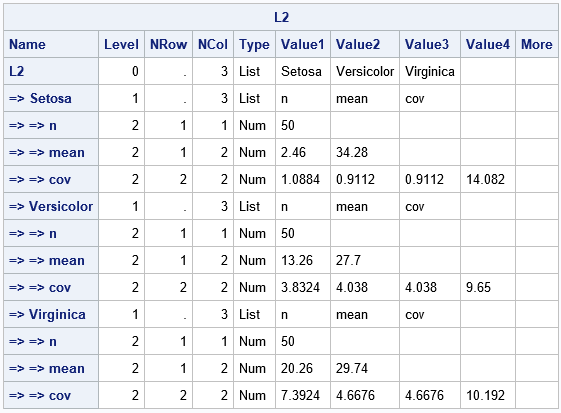The output from the STRUCT call gives a hierarchical overview of the L2 list. The leftmost column indicates the hierarchy of the lists. The list has G=3 items, which are themselves lists. Each list has a name (the group level and m=3 items: the number of observations ('n'), the mean vector ('mean'), and the within-group MLE covariance matrix ('cov'). In this output, notice that there is no need to flatten the covariance matrix into a row vector. For some applications, this list format might be easier to work with. If you want, for example, the mean vector for the 'Setosa' group, you can access it by using the notation m = L2$'Setosa'$'mean'. Or you could extract the 'Setosa' list and access the statistics as follows: SetosaList = L2$'Setosa'; /* get the list of statistics for the 'Setosa' group */ m = SetosaList$'mean'; /* get the mean vector */ C = SetosaList$'cov'; /* get the MLE covariance matrix */ print m[c=varNames], C[c=varNames r=varNames];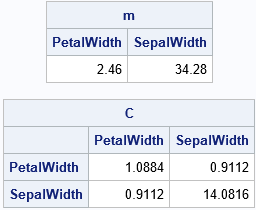### Summary

• How to use the UNIQUE-LOC technique to compute multivariate statistics for each group in the data.
• How to return those statistics by using SAS/IML lists. Two ways to organize the output are presented. The simplest method is to stack the statistics into matrices where the k_th row represents the k_th group. You can then return a list of the matrices. The second method is to return a list of sublists, where the k_th sublist contains the statistics for the k_th list. The first method requires that you "flatten" matrices into row vectors whereas the second method does not.

The post Compute within-group multivariate statistics and store them in a list appeared first on The DO Loop.The multivariate normal distribution is used frequently in multivariate statistics and machine learning. In many applications, you need to evaluate the log-likelihood function in order to compare how well different models fit the data. The log-likelihood for a vector x is the natural logarithm of the multivariate normal (MVN) density function evaluated at x. A probability density function is usually abbreviated as PDF, so the log-density function is also called a log-PDF. This article discusses how to efficiently evaluate the log-likelihood function and the log-PDF. Examples are provided by using the SAS/IML matrix language.

### The multivariate normal PDF

A previous article provides examples of using the LOGPDF function in SAS for univariate distributions. Multivariate distributions are more complicated and are usually written by using matrix-vector notation. The multivariate normal distribution in dimension d has two parameters: A d-dimensional mean vector μ and a d x d covariance matrix Σ. The MVN PDF evaluated at a d-dimensional vector x is
$f(\mathbf{x})= \frac{1}{\sqrt { (2\pi)^d|\boldsymbol \Sigma| } } \exp\left(-\frac{1}{2} (\mathbf{x}-\boldsymbol\mu)^{\rm T} \boldsymbol\Sigma^{-1} ({\mathbf x}-\boldsymbol\mu)\right)$
where |Σ| is the determinant of Σ. I have previously shown how to evaluate the MVN density in the SAS/IML language, and I noted that the argument to the EXP function involves the expression MD(x; μ, Σ)2 = (x-μ)TΣ-1(x-μ), where MD is the Mahalanobis distance between the point x and the mean vector μ.

### Evaluate the MVN log-likelihood function

When you take the natural logarithm of the MVN PDF, the EXP function goes away and the expression becomes the sum of three terms:
$\log(f(\mathbf{x})) = -\frac{1}{2} [ d \log(2\pi) + \log(|\boldsymbol \Sigma|) + {\rm MD}(\mathbf{x}; \boldsymbol\mu, \boldsymbol\Sigma)^2 ]$
The first term in the brackets is easy to evaluate, but the second and third terms appear more daunting. Fortunately, the SAS/IML language provides two functions that simplify the evaluation:

• The LOGABSDET function computes the logarithm of the absolute value of the determinant of a matrix. For a full-rank covariance matrix the determinant is always positive, so the SAS/IML function LogAbsDet(C) returns the log-determinant of a covariance matrix, C.
• The MAHALANOBIS function in SAS/IML evaluates the Mahalanobis distance. The function is vectorized, which means that you can pass in a matrix that has d columns, and the MAHALANOBIS function will return the distance for each row of the matrix.

Some researchers use -2*log(f(x)) instead of log(f(x)) as a measure of likelihood. You can see why: The -2 cancels with the -1/2 in the formula and makes the values positive instead of negative.

### Log likelihood versus log-PDF

I use the terms log-likelihood function and log-PDF function interchangeably, but there is a subtle distinction. The log-PDF is a function of x when the parameters are specified (fixed). The log-likelihood is a function of the parameters when the data are specified. The SAS/IML function in the next section can be used for either purpose. (Note: Some references use the term "log likelihood" to refer only to the sum of the log-PDF scores evaluated at each observation in the sample.)

### Example: Compare the log likelihood values for different parameter values

The log-likelihood function has many applications, but one is to determine whether one model fits the data better than another model. The log likelihood depends on the mean vector μ and the covariance matrix, Σ, which are the parameters for the MVN distribution.

Suppose you have some data that you think are approximately multivariate normal. You can use the log-likelihood function to evaluate whether the model MVN(μ1, Σ1) fits the data better than an alternative model MVN(μ2, Σ2). For example, the Fisher Iris data for the SepalLength and SepalWidth variables appear to be approximately bivariate normal and positively correlated, as shown in the following graph:

title "Iris Data and 95% Prediction Ellipse"; title2 "Assuming Multivariate Normality"; proc sgplot data=Sashelp.Iris noautolegend; where species="Setosa"; scatter x=SepalLength y=SepalWidth / jitter; ellipse x=SepalLength y=SepalWidth; run;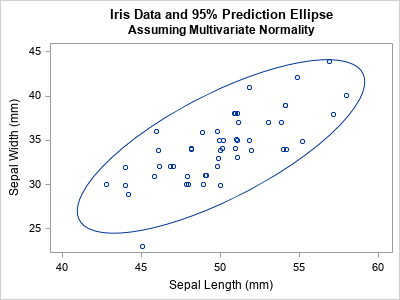The following SAS/IML function defines a function (LogPdfMVN) that evaluates the log-PDF at every observation of a data matrix, given the MVN parameters (or estimates for the parameters). To test the function, the program creates a data matrix from the SepalLength and SepalWidth variables for the observations for which Species="Setosa". The program uses the MEAN and COV functions to compute the maximum likelihood estimates for the data, then calls the LogPdfMVN function to evaluate the log-PDF at each observation:

proc iml; /* This function returns the log-PDF for a MVN(mu, Sigma) density at each row of X. The output is a vector with the same number of rows as X. */ start LogPdfMVN(X, mu, Sigma); d = ncol(X); log2pi = log( 2*constant('pi') ); logdet = logabsdet(Sigma); /* sign of det(Sigma) is '+' */ MDsq = mahalanobis(X, mu, Sigma)##2; /* (x-mu)*inv(Sigma)*(x-mu) */ Y = -0.5 *( MDsq + d*log2pi + logdet ); /* log-PDF for each obs. Sum it for LL */ return( Y ); finish;   /* read the iris data for the Setosa species */ use Sashelp.Iris where(species='Setosa'); read all var {'SepalLength' 'SepalWidth'} into X; close;   n = nrow(X); /* assume no missing values */ m = mean(X); /* maximum likelihood estimate of mu */ S = (n-1)/n * cov(X); /* maximum likelihood estimate of Sigma */ /* evaluate the log likelihood for each observation */ LL = LogPdfMVN(X, m, S);

Notice that you can find the maximum likelihood estimates (m and S) by using a direct computation. For MVN models, you do not need to run a numerical optimization, which is one reason why MVN models are so popular.

The LogPdfMVN function returns a vector that has the same number of rows as the data matrix. The value of the i_th element is the log-PDF of the i_th observation, given the parameters. Because the parameters for the LogPdfMVN function are the maximum likelihood estimates, the total log likelihood (the sum of the LL vector) should be as large as possible for this data. In other words, if we choose different values for μ and Σ, the total log likelihood will be less. Let's see if that is true for this example. Let's change the mean vector and use a covariance matrix that incorrectly postulates that the SepalLength and SepalWidth variables are negatively correlated. The following statements compute the log likelihood for the alternative model:

/* What if we use "wrong" parameters? */ m2 = {45 30}; S2 = {12 -10, -10 14}; /* this covariance matrix indicates negative correlation */ LL_Wrong = LogPdfMVN(X, m2, S2); /* LL for each obs of the alternative model */   /* The total log likelihood is sum(LL) over all obs */ TotalLL = sum(LL); TotalLL_Wrong = sum(LL_Wrong); print TotalLL TotalLL_Wrong;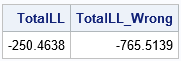As expected, the total log likelihood is larger for the first model than for the second model. The interpretation that the first model fits the data better than the second model.

Although the total log likelihood (the sum) is often used to choose the better model, the log-PDF of the individual observations are also important. The individual log-PDF values identify which observations are unlikely to come from a distribution with the given parameters.

### Visualize the log-likelihood for each model

The easiest way to demonstrate the difference between the "good" and "bad" model parameters is to draw the bivariate scatter plot of the data and color each observation by the log-PDF at that position.

The plot for the first model (which fits the data well) is shown below. The observations are colored by the log-PDF value (the LL vector) for each observation. Most observations are blue or blue-green because those colors indicate high values of the log-PDF.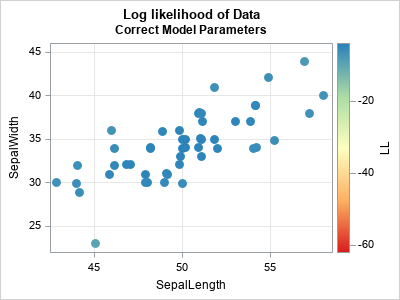The plot for the second model (which intentionally misspecifies the parameters) is shown below. The observations near (45, 30) are blue or blue-green because that is the location of the specified mean parameter. A prediction ellipse for the specified model has a semimajor axis that slopes from the upper left to the lower right. Therefore, the points in the upper right corner of the plot have a large Mahalanobis distance and a very negative log-PDF. These points are colored yellow, orange, or red. They are "outliers" in the sense that they are unlikely to be observed in a random sample from an MVN distribution that has the second set of parameters.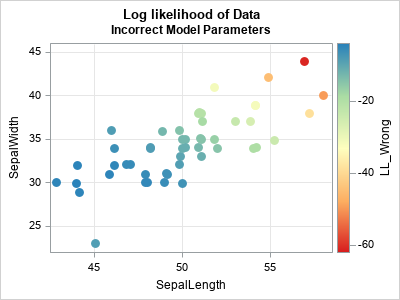### What is a "large" log-PDF value?

For this example, the log-PDF is negative for each observation, so "large" and "small" can be confusing terms. I want to emphasize two points:

1. When I say a log-PDF value is "large" or "high," I mean "close to the maximum value of the log-PDF function." For example, -3.1 is a large log-PDF value for these data. Observations that are far from the mean vector are very negative. For example, -40 is a "very negative" value.
2. The maximum value of the log-PDF occurs when an observation exactly equals the mean vector. Thus the log-PDF will never be larger than -0.5*( d*log(2π) + log(det(Σ)) ). For these data, the maximum value of the log-PDF is -4.01 when you use the maximum likelihood estimates as MVN parameters.

### Summary

In summary, this article shows how to evaluate the log-PDF of the multivariate normal distribution. The log-PDF values indicate how likely each observation would be in a random sample, given parameters for an MVN model. If you sum the log-PDF values over all observations, you get a statistic (the total log likelihood) that summarizes how well a model fits the data. If you are comparing two models, the one with the larger log likelihood is the model that fits better.

The post How to evaluate the multivariate normal log likelihood appeared first on The DO Loop.The multivariate normal distribution is used frequently in multivariate statistics and machine learning. In many applications, you need to evaluate the log-likelihood function in order to compare how well different models fit the data. The log-likelihood for a vector x is the natural logarithm of the multivariate normal (MVN) density function evaluated at x. A probability density function is usually abbreviated as PDF, so the log-density function is also called a log-PDF. This article discusses how to efficiently evaluate the log-likelihood function and the log-PDF. Examples are provided by using the SAS/IML matrix language.

### The multivariate normal PDF

A previous article provides examples of using the LOGPDF function in SAS for univariate distributions. Multivariate distributions are more complicated and are usually written by using matrix-vector notation. The multivariate normal distribution in dimension d has two parameters: A d-dimensional mean vector μ and a d x d covariance matrix Σ. The MVN PDF evaluated at a d-dimensional vector x is
$f(\mathbf{x})= \frac{1}{\sqrt { (2\pi)^d|\boldsymbol \Sigma| } } \exp\left(-\frac{1}{2} (\mathbf{x}-\boldsymbol\mu)^{\rm T} \boldsymbol\Sigma^{-1} ({\mathbf x}-\boldsymbol\mu)\right)$
where |Σ| is the determinant of Σ. I have previously shown how to evaluate the MVN density in the SAS/IML language, and I noted that the argument to the EXP function involves the expression MD(x; μ, Σ)2 = (x-μ)TΣ-1(x-μ), where MD is the Mahalanobis distance between the point x and the mean vector μ.

### Evaluate the MVN log-likelihood function

When you take the natural logarithm of the MVN PDF, the EXP function goes away and the expression becomes the sum of three terms:
$\log(f(\mathbf{x})) = -\frac{1}{2} [ d \log(2\pi) + \log(|\boldsymbol \Sigma|) + {\rm MD}(\mathbf{x}; \boldsymbol\mu, \boldsymbol\Sigma)^2 ]$
The first term in the brackets is easy to evaluate, but the second and third terms appear more daunting. Fortunately, the SAS/IML language provides two functions that simplify the evaluation:

• The LOGABSDET function computes the logarithm of the absolute value of the determinant of a matrix. For a full-rank covariance matrix the determinant is always positive, so the SAS/IML function LogAbsDet(C) returns the log-determinant of a covariance matrix, C.
• The MAHALANOBIS function in SAS/IML evaluates the Mahalanobis distance. The function is vectorized, which means that you can pass in a matrix that has d columns, and the MAHALANOBIS function will return the distance for each row of the matrix.

Some researchers use -2*log(f(x)) instead of log(f(x)) as a measure of likelihood. You can see why: The -2 cancels with the -1/2 in the formula and makes the values positive instead of negative.

### Log likelihood versus log-PDF

I use the terms log-likelihood function and log-PDF function interchangeably, but there is a subtle distinction. The log-PDF is a function of x when the parameters are specified (fixed). The log-likelihood is a function of the parameters when the data are specified. The SAS/IML function in the next section can be used for either purpose. (Note: Some references use the term "log likelihood" to refer only to the sum of the log-PDF scores evaluated at each observation in the sample.)

### Example: Compare the log likelihood values for different parameter values

The log-likelihood function has many applications, but one is to determine whether one model fits the data better than another model. The log likelihood depends on the mean vector μ and the covariance matrix, Σ, which are the parameters for the MVN distribution.

Suppose you have some data that you think are approximately multivariate normal. You can use the log-likelihood function to evaluate whether the model MVN(μ1, Σ1) fits the data better than an alternative model MVN(μ2, Σ2). For example, the Fisher Iris data for the SepalLength and SepalWidth variables appear to be approximately bivariate normal and positively correlated, as shown in the following graph:

title "Iris Data and 95% Prediction Ellipse"; title2 "Assuming Multivariate Normality"; proc sgplot data=Sashelp.Iris noautolegend; where species="Setosa"; scatter x=SepalLength y=SepalWidth / jitter; ellipse x=SepalLength y=SepalWidth; run;The following SAS/IML function defines a function (LogPdfMVN) that evaluates the log-PDF at every observation of a data matrix, given the MVN parameters (or estimates for the parameters). To test the function, the program creates a data matrix from the SepalLength and SepalWidth variables for the observations for which Species="Setosa". The program uses the MEAN and COV functions to compute the maximum likelihood estimates for the data, then calls the LogPdfMVN function to evaluate the log-PDF at each observation:

proc iml; /* This function returns the log-PDF for a MVN(mu, Sigma) density at each row of X. The output is a vector with the same number of rows as X. */ start LogPdfMVN(X, mu, Sigma); d = ncol(X); log2pi = log( 2*constant('pi') ); logdet = logabsdet(Sigma); /* sign of det(Sigma) is '+' */ MDsq = mahalanobis(X, mu, Sigma)##2; /* (x-mu)*inv(Sigma)*(x-mu) */ Y = -0.5 *( MDsq + d*log2pi + logdet ); /* log-PDF for each obs. Sum it for LL */ return( Y ); finish;   /* read the iris data for the Setosa species */ use Sashelp.Iris where(species='Setosa'); read all var {'SepalLength' 'SepalWidth'} into X; close;   n = nrow(X); /* assume no missing values */ m = mean(X); /* maximum likelihood estimate of mu */ S = (n-1)/n * cov(X); /* maximum likelihood estimate of Sigma */ /* evaluate the log likelihood for each observation */ LL = LogPdfMVN(X, m, S);

Notice that you can find the maximum likelihood estimates (m and S) by using a direct computation. For MVN models, you do not need to run a numerical optimization, which is one reason why MVN models are so popular.

The LogPdfMVN function returns a vector that has the same number of rows as the data matrix. The value of the i_th element is the log-PDF of the i_th observation, given the parameters. Because the parameters for the LogPdfMVN function are the maximum likelihood estimates, the total log likelihood (the sum of the LL vector) should be as large as possible for this data. In other words, if we choose different values for μ and Σ, the total log likelihood will be less. Let's see if that is true for this example. Let's change the mean vector and use a covariance matrix that incorrectly postulates that the SepalLength and SepalWidth variables are negatively correlated. The following statements compute the log likelihood for the alternative model:

/* What if we use "wrong" parameters? */ m2 = {45 30}; S2 = {12 -10, -10 14}; /* this covariance matrix indicates negative correlation */ LL_Wrong = LogPdfMVN(X, m2, S2); /* LL for each obs of the alternative model */   /* The total log likelihood is sum(LL) over all obs */ TotalLL = sum(LL); TotalLL_Wrong = sum(LL_Wrong); print TotalLL TotalLL_Wrong;As expected, the total log likelihood is larger for the first model than for the second model. The interpretation that the first model fits the data better than the second model.

Although the total log likelihood (the sum) is often used to choose the better model, the log-PDF of the individual observations are also important. The individual log-PDF values identify which observations are unlikely to come from a distribution with the given parameters.

### Visualize the log-likelihood for each model

The easiest way to demonstrate the difference between the "good" and "bad" model parameters is to draw the bivariate scatter plot of the data and color each observation by the log-PDF at that position.

The plot for the first model (which fits the data well) is shown below. The observations are colored by the log-PDF value (the LL vector) for each observation. Most observations are blue or blue-green because those colors indicate high values of the log-PDF.The plot for the second model (which intentionally misspecifies the parameters) is shown below. The observations near (45, 30) are blue or blue-green because that is the location of the specified mean parameter. A prediction ellipse for the specified model has a semimajor axis that slopes from the upper left to the lower right. Therefore, the points in the upper right corner of the plot have a large Mahalanobis distance and a very negative log-PDF. These points are colored yellow, orange, or red. They are "outliers" in the sense that they are unlikely to be observed in a random sample from an MVN distribution that has the second set of parameters.### What is a "large" log-PDF value?

For this example, the log-PDF is negative for each observation, so "large" and "small" can be confusing terms. I want to emphasize two points:

1. When I say a log-PDF value is "large" or "high," I mean "close to the maximum value of the log-PDF function." For example, -3.1 is a large log-PDF value for these data. Observations that are far from the mean vector are very negative. For example, -40 is a "very negative" value.
2. The maximum value of the log-PDF occurs when an observation exactly equals the mean vector. Thus the log-PDF will never be larger than -0.5*( d*log(2π) + log(det(Σ)) ). For these data, the maximum value of the log-PDF is -4.01 when you use the maximum likelihood estimates as MVN parameters.

### Summary

In summary, this article shows how to evaluate the log-PDF of the multivariate normal distribution. The log-PDF values indicate how likely each observation would be in a random sample, given parameters for an MVN model. If you sum the log-PDF values over all observations, you get a statistic (the total log likelihood) that summarizes how well a model fits the data. If you are comparing two models, the one with the larger log likelihood is the model that fits better.

The post How to evaluate the multivariate normal log likelihood appeared first on The DO Loop.A previous article discusses the pooled variance for two or groups of univariate data. The pooled variance is often used during a t test of two independent samples. For multivariate data, the analogous concept is the pooled covariance matrix, which is an average of the sample covariance matrices of the groups. If you assume that the covariances within the groups are equal, the pooled covariance matrix is an estimate of the common covariance. This article shows how to compute and visualize a pooled covariance matrix in SAS. It explains how the pooled covariance relates to the within-group covariance matrices. It discusses a related topic, called the between-group covariance matrix.

The within-group matrix is sometimes called the within-class covariance matrix because a classification variable is used to identify the groups. Similarly, the between-group matrix is sometimes called the between-class covariance matrix.

### Visualize within-group covariances

Suppose you want to analyze the covariance in the groups in Fisher's iris data (the Sashelp.Iris data set in SAS). The data set contains four numeric variables, which measure the length and width of two flower parts, the sepal and the petal. Each observation is for a flower from an iris species: Setosa, Versicolor, or Virginica. The Species variable in the data identifies observations that belong to each group, and each group has 50 observations. The following call to PROC SGPLOT creates two scatter plots and overlays prediction ellipses for two pairs of variables:

title "68% Prediction Ellipses for Iris Data"; proc sgplot data=Sashelp.Iris; scatter x=SepalLength y=SepalWidth / group=Species transparency=0.5; ellipse x=SepalLength y=SepalWidth / group=Species alpha=0.32 lineattrs=(thickness=2); run; proc sgplot data=Sashelp.Iris; scatter x=PetalLength y=PetalWidth / group=Species transparency=0.5; ellipse x=PetalLength y=PetalWidth / group=Species alpha=0.32 lineattrs=(thickness=2); run;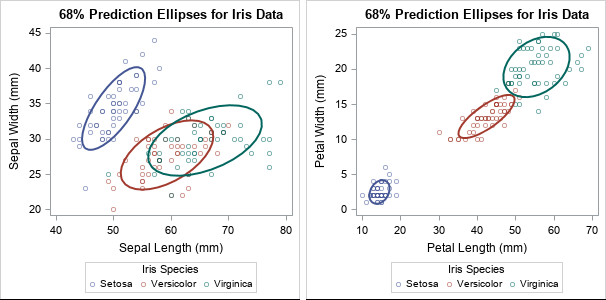The ellipses enable you to visually investigate whether the variance of the data within the three groups appears to be the same. For these data, the answer is no because the ellipses have different shapes and sizes. Some of the prediction ellipses have major axes that are oriented more steeply than others. Some of the ellipses are small, others are relatively large.

You might wonder why the graph shows a 68% prediction ellipse for each group. Recall that prediction ellipses are a multivariate generalization of "units of standard deviation." If you assume that measurements in each group are normally distributed, 68% of random observations are within one standard deviation from the mean. So for multivariate normal data, a 68% prediction ellipse is analogous to +/-1 standard deviation from the mean.

### The pooled covariance is an average of within-group covariances

The pooled covariance is used in linear discriminant analysis and other multivariate analyses. It combines (or "pools") the covariance estimates within subgroups of data. The pooled covariance is one of the methods used by Friendly and Sigal (TAS, 2020) to visualize homogeneity tests for covariance matrices.

Suppose you collect multivariate data for $k$ groups and $S_i$ is the sample covariance matrix for the $n_i$ observations within the $i$th group. If you believe that the groups have a common variance, you can estimate it by using the pooled covariance matrix, which is a weighted average of the within-group covariances:
$S_p = \Sigma_{i=1}^k (n_i-1)S_i / \Sigma_{i=1}^k (n_i - 1)$

If all groups have the same number of observations, then the formula simplifies to $\Sigma_{i=1}^k S_i / k$, which is the simple average of the matrices. If the group sizes are different, then the pooled variance is a weighted average, where larger groups receive more weight than smaller groups.

### Compute the pooled covariance in SAS

In SAS, you can often compute something in two ways. The fast-and-easy way is to find a procedure that does the computation. A second way is to use the SAS/IML language to compute the answer yourself. When I compute something myself (and get the same answer as the procedure!), I increase my understanding.

Suppose you want to compute the pooled covariance matrix for the iris data. The fast-and-easy way to compute a pooled covariance matrix is to use PROC DISCRIM. The procedure supports the OUTSTAT= option, which writes many multivariate statistics to a data set, including the within-group covariance matrices, the pooled covariance matrix, and something called the between-group covariance. (It also writes analogous quantities for centered sum-of-squares and crossproduct (CSSCP) matrices and for correlation matrices.)

proc discrim data=sashelp.iris method=normal pool=yes outstat=Cov noprint; class Species; var SepalLength SepalWidth PetalLength PetalWidth; run;   proc print data=Cov noobs; where _TYPE_ = "PCOV"; format _numeric_ 6.2; var _TYPE_ _NAME_ Sepal: Petal:; run;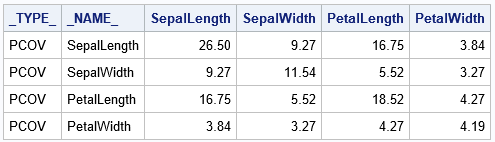The table shows the "average" covariance matrix, where the average is across the three species of flowers.

### Within-group covariance matrices

The same output data set contains the within-group and the between-group covariance matrices. The within-group matrices are easy to understand. They are the covariance matrices for the observations in each group. Accordingly, there are three such matrices for these data: one for the observations where Species="Setosa", one for Species="Versicolor", and one for Species="Virginica". The following call to PROC PRINT displays the three matrices:

proc print data=Cov noobs; where _TYPE_ = "COV" and Species^=" "; format _numeric_ 6.2; run;

The output is not particularly interesting, so it is not shown. The matrices are the within-group covariances that were visualized earlier by using prediction ellipses.

### Visual comparison of the pooled covariance and the within-group covariance

Friendly and Sigal (2020, Figure 1) overlay the prediction ellipses for the pooled covariance on the prediction ellipses for the within-group covariances. A recreation of Figure 1 in SAS is shown below. You can use the SAS/IML language to draw prediction ellipses from covariance matrices.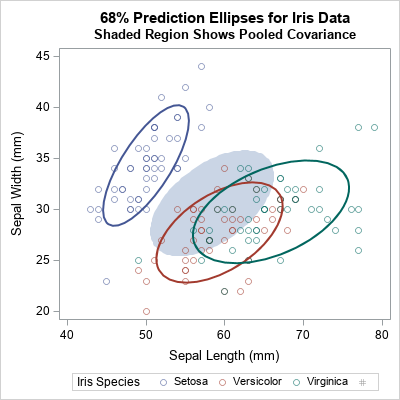The shaded region is the prediction ellipse for these two variables in the pooled covariance matrix. It is centered at the weighted average of the group means. You can see that the pooled ellipse looks like an average of the other ellipses. This graph shows only one pair of variables, but see Figure 2 of Friendly and Sigal (2020) for a complete scatter plot matrix that compares the pooled covariance to the within-group covariance for each pair of variables.

### Between-group covariance matrices

Another matrix in the PROC DISCRIM output is the so-called between-group covariance matrix. Intuitively, the between-group covariance matrix is related to the difference between the full covariance matrix of the data (where the subgroups are ignored) and the pooled covariance matrix (where the subgroups are averaged). The precise definition is given in the next section. For now, here is how to print the between-group covariance matrix from the output of PROC DISCRIM:

proc print data=Cov noobs; where _TYPE_ = "BCOV"; format _numeric_ 6.2; run;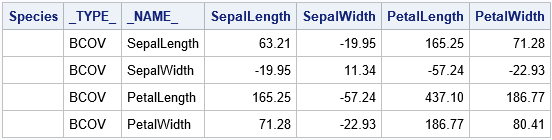### How to compute the pooled and between-group covariance

If I can compute a quantity "by hand," then I know that I truly understand it. Thus, I wrote a SAS/IML program that reproduces the computations made by PROC DISCRIM. The following steps are required to compute each of these matrices from first principles.

1. For each group, compute the covariance matrix (S_i) of the observations in that group.
2. Note that the quantity (n_i - 1)*S_i is the centered sum-of-squares and crossproducts (CSSCP) matrix for the group. Let M be the sum of the CSSCP matrices. The sum is the numerator for the pooled covariance.
3. Form the pooled covariance matrix as S_p = M / (N-k).
4. Let C be the CSSCP data for the full data (which is (N-1)*(Full Covariance)). The between-group covariance matrix is BCOV = (C - M) * k / (N*(k-1)).

You can use the UNIQUE-LOC trick to iterate over the data for each group. The following SAS/IML program implements these computations:

/* Compute a pooled covariance matrix when observations belong to k groups with sizes n1, n2, ..., nk, where n1+n2+...+nk = N */ proc iml; varNames = {'SepalLength' 'SepalWidth' 'PetalLength' 'PetalWidth'}; use Sashelp.iris; read all var varNames into Z; read all var "Species" into Group; close;   /* assume complete cases, otherwise remove rows with missing values */ N = nrow(Z);   /* compute the within-group covariance, which is the covariance for the observations in each group */ u = unique(Group); k = ncol(u); /* number of groups */ p = ncol(varNames); /* number of variables */ M = j(p, p, 0); /* sum of within-group CSCCP matrices */ do i = 1 to k; idx = loc(Group = u[i]); /* find rows for this group */ X = Z[idx,]; /* extract obs for i_th group */ n_i = nrow(X); /* n_i = size of i_th group */ S = cov(X); /* within-group cov */ /* accumulate the weighted sum of within-group covariances */ M = M + (n_i-1) * S; /* (n_i-1)*S is centered X*X */ end;   /* The pooled covariance is an average of the within-class covariance matrices. */ Sp = M / (N-k); print Sp[L="Pooled Cov" c=varNames r=VarNames format=6.2];   /* The between-class CSSCP is the difference between total CSSCP and the sum of the within-group CSSCPs. The SAS doc for PROC DISCRIM defines the between-class covariance matrix as the between-class SSCP matrix divided by N*(k-1)/k, where N is the number of observations and k is the number of classes. */ /* the total covariance matrix ignores the groups */ C = (N-1)*cov(Z); BCSSCP = C - M; /* between = Full - Sum(Within) */ BCov = BCSSCP * k/( N*(k-1) ); print BCov[L="Between Cov" c=varNames r=VarNames format=6.2];`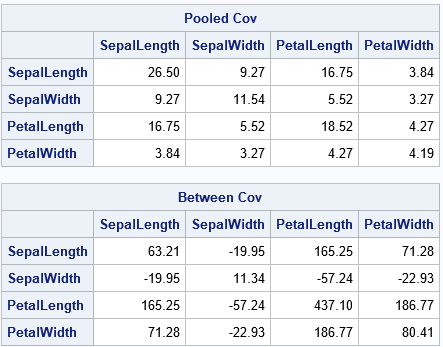Success! The SAS/IML program shows the computations that are needed to reproduce the pooled and between-group covariance matrices. The results are the same as are produced by PROC DISCRIM.

### Summary

In multivariate ANOVA, you might assume that the within-group covariance is constant across different groups in the data. The pooled covariance is an estimate of the common covariance. It is a weighted average of the sample covariances for each group, where the larger groups are weighted more heavily than smaller groups. I show how to visualize the pooled covariance by using prediction ellipses.

You can use PROC DISCRIM to compute the pooled covariance matrix and other matrices that represent within-group and between-group covariance. I also show how to compute the matrices from first principles by using the SAS/IML language. You can download the SAS program that performs the computations and creates the graphs in this article.

The post Pooled, within-group, and between-group covariance matrices appeared first on The DO Loop.